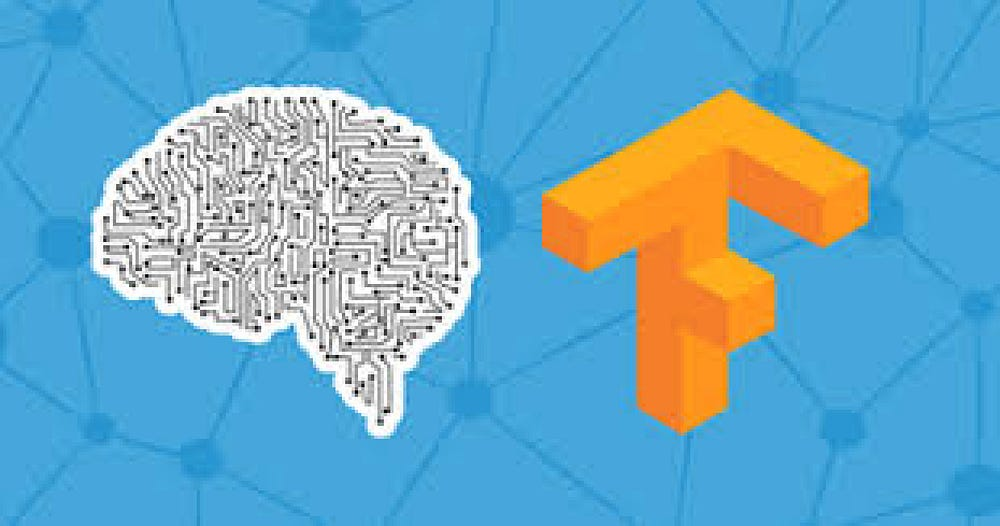# Get started with TensorFlow and Deep Learning Part-1

Source: Deep Learning on Medium# Hello Neural Networks

To show how that works, let’s take a look at a set of numbers and see if you can determine the pattern between them. Okay, here are the numbers.

x = -1, 0, 1, 2, 3, 4

y = -3, -1, 1, 3, 5, 7

You easily figure this out `y = 2x — 1`

You probably tried that out with a couple of other values and see that it fits. Congratulations, you’ve just done the basics of machine learning in your head.

Okay, here’s our first line of code. This is written using Python and TensorFlow and an API in TensorFlow called keras. Keras makes it really easy to define neural networks. A neural network is basically a set of functions which can learn patterns.

`model = keras.Sequential([keras.layers.Dense(units = 1, input_shape = )])`

The simplest possible neural network is one that has only one neuron in it, and that’s what this line of code does. In keras we use the word `Dense` to define a layer of connected neurons. There is only one `Dense` here means that there is only one layer and there is only single unit in it so there is only one neuron. Successive layers in keras are defined in a sequence so the word `Sequential` . You define the shape of what’s input to the neural network in the first and in this case the only layer, and you can see that our input shape is super simple. It’s just one value. You’ve probably seen that for machine learning, you need to know and use a lot of math, calculus probability and the like. It’s really good to understand that as you want to optimize your models but the nice thing for now about TensorFlow and keras is that a lot of that math is implemented for you in functions. There are two function roles that you should be aware of though and these are loss functions and optimizers.

`model.compile(optimizer = 'sgd', loss = 'mean_squared_error')`

This lines defines that for us. So, lets understand what it is.

Understand it like this the neural network has no idea of the relation between X and Y so it makes a random guess say `y=x+3` . It will then use the data that it knows about, that’s the set of Xs and Ys that we’ve already seen to measure how good or how bad its guess was. The loss function measures this and then gives the data to the optimizer which figures out the next guess. So the optimizer thinks about how good or how badly the guess was done using the data from the loss function. Then the logic is that each guess should be better than the one before. As the guesses get better and better, an accuracy approaches 100 percent, the term convergence is used.

Here we have used the loss function as mean squared error and optimizer as SGD or stochactic gradient descent.

Our next step is to represent the known data. These are the Xs and the Ys that you saw earlier. The np.array is using a Python library called numpy that makes data representation particularly enlists much easier. So here you can see we have one list for the Xs and another one for the Ys. The training takes place in the fit command. Here we’re asking the model to figure out how to fit the X values to the Y values. The epochs equals 500 value means that it will go through the training loop 500 times. This training loop is what we described earlier. Make a guess, measure how good or how bad the guesses with the loss function, then use the optimizer and the data to make another guess and repeat this. When the model has finished training, it will then give you back values using the predict method.

Here’s the code of what we talked about.

`xs = np.array([-1.0, 0.0, 1.0, 2.0, 3.0, 4.0], dtype=float)ys = np.array([-3.0, -1.0, 1.0, 3.0, 5.0, 7.0], dtype=float)model.fit(xs, ys, epochs=500)print(model.predict([10.0]))`

So, what output do you except — 19, right?

But when you try this in the workbook yourself you will see it gives me a value close to 19 not 19. Like you might receive-

`[[18.987219]]`

Why do you think this happens because the equation is `y = 2x-1` .

There are two main reasons:

The first is that you trained it using very little data. There’s only six points. Those six points are linear but there’s no guarantee that for every X, the relationship will be Y equals 2X minus 1. There’s a very high probability that Y equals 19 for X equals 10, but the neural network isn’t positive. So it will figure out a realistic value for Y. The the second main reason. When using neural networks, as they try to figure out the answers for everything, they deal in probability. You’ll see that a lot and you’ll have to adjust how you handle answers to fit. Keep that in mind as you work through the code. Okay, enough talking. Now let’s get hands-on and write the code that we just saw and then we can run it.